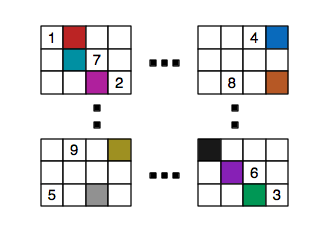# Unfriendly neighbours

Probability Level 5Find the greatest positive integer $N$, such that for any arrangement of the positive integers $1, 2, 3, \ldots , 1600$ in a $40 \times 40$ table, there exists two numbers located in the same row or in the same column such that the (positive) difference of these numbers is not less than $N$.

×